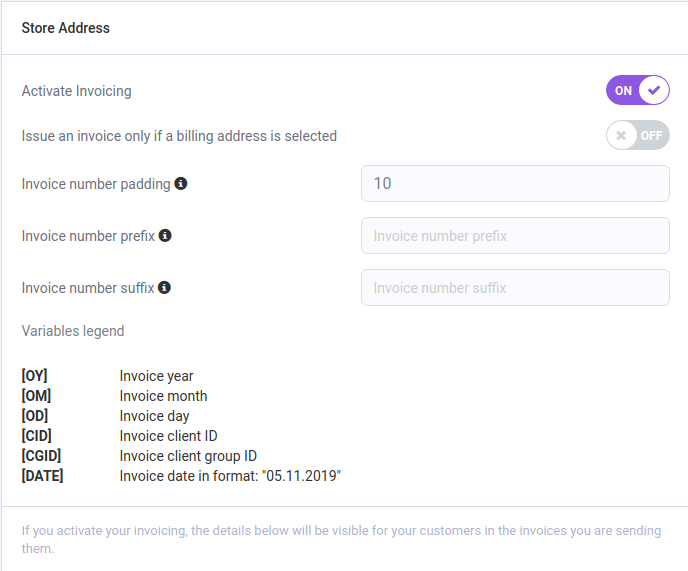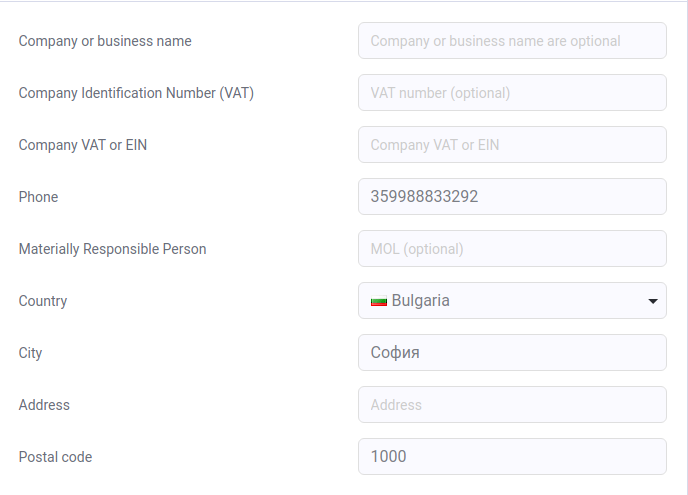Invoice information

Go to your control panel > Settings > Cart settings > Store Address1.  You can choose whether to enable Activating Invoicing from the On / Off button.

2.  Issue an invoice only if a billing address is selected - Self-explanatory option, which you can choose to enable/disable in accordance with your needs.

3.  Invoice number padding - This option determines how many digits would be filled with the number 0. The default is 10.

Example:

*  If the invoice is number 1, 9 zeros will be automatically added.

*  If the invoice number is 123 and you have filled in 10, then the number will look like this: 0000000123.

*  If you set fill 5 and the number is 123456, then the number will look like this: 123456, even if the invoice number is larger than the preset fill number, it will be displayed in full.

4.  Invoice number prefix - This is the invoice number respectively.

Example:

*  If the invoice number is 123 and created in 2019, and you set the [OY] prefix, then the number will look like this: 2019-123.

5.  Invoice number suffix - This is the opposite of the Prefix, the number with which the invoice ends.

Example:

*  If the invoice number is 123 and was created in 2019 and you set a suffix - [OY], then the number will look like this: 123-2019.

Company information